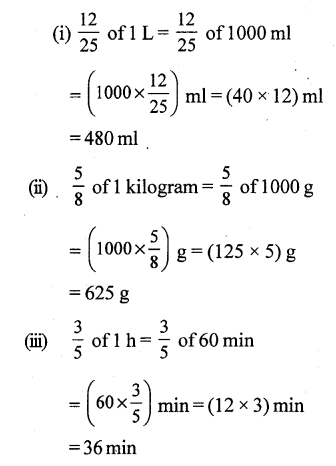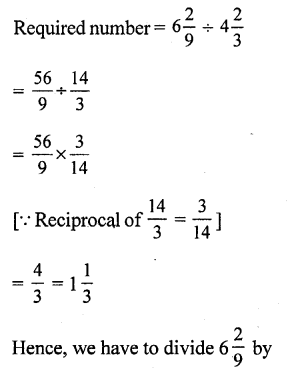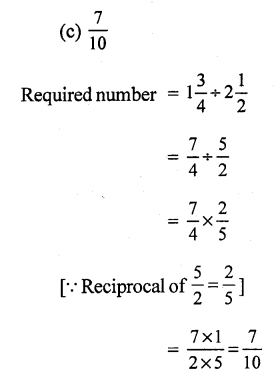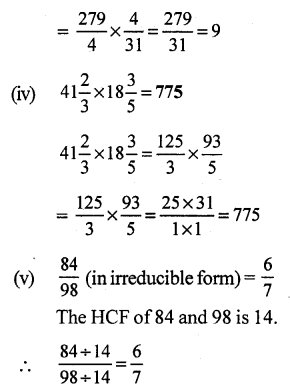# RS Aggarwal Solutions Class 7 Chapter 2 Fractions CCE Test Paper

In this chapter, we provide RS Aggarwal Solutions for Class 7 Chapter 2 Fractions CCE Test Paper for English medium students, Which will very helpful for every student in their exams. Students can download the latest RS Aggarwal Solutions for Class 7 Chapter 2 Fractions CCE Test Paper Maths pdf, free RS Aggarwal Solutions Class 7 Chapter 2 Fractions CCE Test Paper Maths book pdf download. Now you will get step by step solution to each question.

### RS Aggarwal Class 7 Solutions Chapter 2 Fractions CCE Test Paper Download PDF

Question 1.
Solution:
(i) A number of the form ab, where a and b are rational numbers, is called a natural number.
Here, a is the numerator and b is the denominator.
(a) 2/3 is a fraction with 2 as the numerator and 3 as the denominator.
(b) 1/25 is a fraction with 12 as the numerator and 5 as the denominator.
(ii) A fraction whose denominator is a whole number other than 10, 100, 1000, etc., is called a vulgar faction.
Examples : 2/5 and 4/15.
(iii) A fraction whose numerator is greater than or equal to its denominator is called an improper fraction.
Example : 1/13 and 41/35

Question 2.
Solution:Hence, the required number is 8/25

Question 3.
Solution:Question 4.
Solution:Question 5.
Solution:Question 6.
Solution:Question 7.
Solution:Question 8.
Solution:Question 9.
Solution:Mark (√) against the correct answer in each of the following:

Question 10.

Solution:
(d) 58
58 is a vulgar fraction, because its denominator is other than 10,100, 1000, etc.

Question 11.
Solution:
(c) 4663
A fraction ab is said to be irreducible or in its lowest terms if the HCF of a and b is 1
46 = 2 x 23 x 1
63 = 3 x 3 x 21 x 1
Clearly, the HCF of 46 and 63 is 1.
Hence, 4663 is an irreducible fraction.

Question 12.
Solution:
(d) None of these
Reciprocal of 135 = Reciprocal of 85 = 58

Question 13.
Solution:Question 14.
Solution:Question 15.
Solution:Question 16.
Solution:
(b) 33 km
Distance covered by the car onQuestion 17.
Solution:Question 18.
Solution:
(i) False.
By cross multiplication, we have:
9 x 24 = 216 and 13 x 16 = 208
However, 216 > 208
916 > 1324All Chapter RS Aggarwal Solutions For Class 7 Maths

—————————————————————————–

All Subject NCERT Exemplar Problems Solutions For Class 7

All Subject NCERT Solutions For Class 7

*************************************************

I think you got complete solutions for this chapter. If You have any queries regarding this chapter, please comment on the below section our subject teacher will answer you. We tried our best to give complete solutions so you got good marks in your exam.

If these solutions have helped you, you can also share rsaggarwalsolutions.in to your friends.